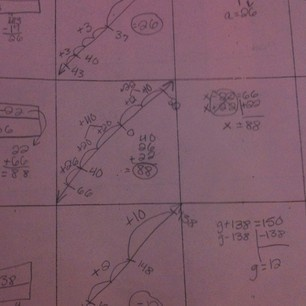# D DuenasThis is a math paper demonstrating multiple ways to solve a math problem. The problem is solving a one-step equation. One method is to use a bar model. You cut a rectangle in half (lengthwise) and put on the bottom half the number you are solving for. The top half has a section labeled x (or variable) and another section with the known value. Students use problem solving to solve for x.

Another method is using a number line to solve an equation. You draw a number line and start a either the known number or what you are solving for. Then, you count forward or backwards to solve for x. Students like to draw the number line at an angle to show either solving by increasing numbers or decreasing numbers.

A third method is a method we call: “Build a Fence.” Students solve equations in a traditional way. But, this method shows how you do the inverse operations on each side of the equation. This keeps the equation, “Balanced.”

My students enjoy learning multiple methods to solve math problems.

0